# How to Find the Day of The Week of a Given Date in Google Sheets

Learn how to use the WEEKDAY and SWITCH functions to find and include the day of the week of any date in a Google Sheet.1. The WEEKDAY function
2. Combining WEEKDAY function with SWITCH function
3. Sample sheet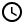If you are handling large datasets with dates, and their corresponding days of the week are found to be important, then you need to automate conversion of these dates to their days of the week. In this tutorial, you will learn two things: the WEEKDAY function and the SWITCH function.

## The WEEKDAY function

The WEEKDAY function analyzes a date and then produces the corresponding day of the week in the form of a number. The function has the following syntax:

=WEEKDAY(date, [type])

By default, the WEEKDAY function starts its count on the Sunday, giving the Sunday a value of 1. Therefore, the value of Saturday is 7. This is set as type=1. As this is the default type of the function, there is no need to specify as such.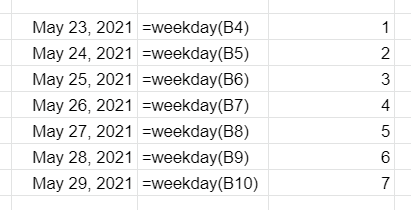The WEEKDAY function applied to various dates. First column contains a list of dates; second column contains the WEEKDAY function applied to the first column; third column contains the result of the WEEKDAY function applied to the first column. Sunday is set as day one in this example.

The WEEKDAY function can also be set up to start counting on Monday instead of Sunday. This means that Monday is set as 1 while Sunday is set as 7. To do so, set type=2:

=WEEKDAY(date, 2)

You can see the result below: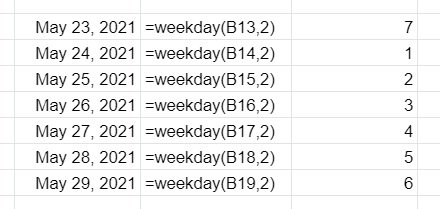The WEEKDAY function applied to various dates. First column contains a list of dates; second column contains the WEEKDAY function applied to the first column; third column contains the result of the WEEKDAY function applied to the first column. Monday is set as the first day of the week in this example.

There is a third type, where Monday is set as 0 while Sunday is set as 6. You can see the result below: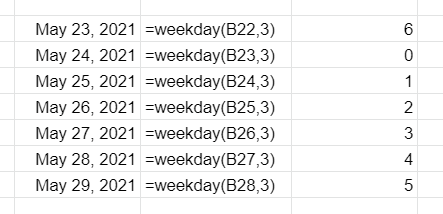The WEEKDAY function applied to various dates. First column contains a list of dates; second column contains the WEEKDAY function applied to the first column; third column contains the result of the WEEKDAY function applied to the first column. Monday is set as the first day of the week in this example, but Monday counts as 0.

## Combining WEEKDAY function with SWITCH function

We can use another function to convert the output of the WEEKDAY function to its corresponding name. For this purpose, we will use the SWITCH function. The SWITCH function is ideal for cases where you only get a set of exact values to convert. The advantage of the SWITCH function over the IF function is that it is more compact and does not require you to add IF functions inside another IF function.

If a function you use outputs one of three possible values, let’s say 1, 2, and 3, and you need to convert them to their corresponding meanings AAA, BBB, and CCC, respectively, you can use the SWITCH function as follows:

=SWITCH(expression,1,”AAA”,2,”BBB”,3,”CCC”)

The expression can either be a formula or a cell reference. We will combine this with the WEEKDAY function. The formula now looks like this:

=switch(WEEKDAY(cell),1,"Sunday",2,"Monday",3,"Tuesday",4,"Wednesday",5, "Thursday",6,"Friday",7,"Saturday")

Change the cell to the cell reference containing the date. Since the WEEKDAY function is nested inside the SWITCH function, you do not need to allot an extra column for the value of the WEEKDAY function. You can check the results below: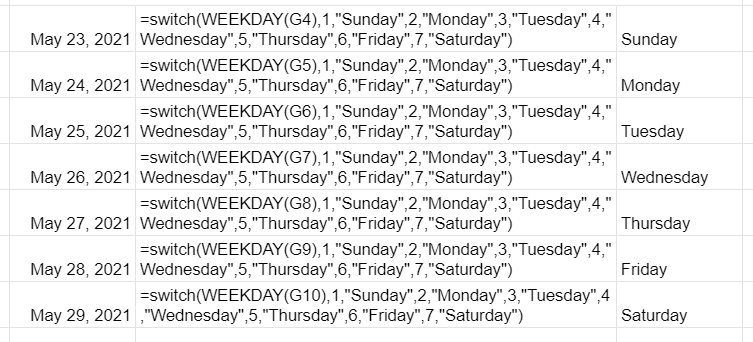The WEEKDAY function combined with the SWITCH function to convert the output of the WEEKDAY function to the names of the days of the week.

## Sample sheet

You can check our sample sheet below:

Oops! Something went wrong while submitting the form.

August 5, 2021If you are handling large datasets with dates, and their corresponding days of the week are found to be important, then you need to automate conversion of these dates to their days of the week. In this tutorial, you will learn two things: the WEEKDAY function and the SWITCH function.

## The WEEKDAY function

The WEEKDAY function analyzes a date and then produces the corresponding day of the week in the form of a number. The function has the following syntax:

=WEEKDAY(date, [type])

By default, the WEEKDAY function starts its count on the Sunday, giving the Sunday a value of 1. Therefore, the value of Saturday is 7. This is set as type=1. As this is the default type of the function, there is no need to specify as such.The WEEKDAY function applied to various dates. First column contains a list of dates; second column contains the WEEKDAY function applied to the first column; third column contains the result of the WEEKDAY function applied to the first column. Sunday is set as day one in this example.

The WEEKDAY function can also be set up to start counting on Monday instead of Sunday. This means that Monday is set as 1 while Sunday is set as 7. To do so, set type=2:

=WEEKDAY(date, 2)

You can see the result below:The WEEKDAY function applied to various dates. First column contains a list of dates; second column contains the WEEKDAY function applied to the first column; third column contains the result of the WEEKDAY function applied to the first column. Monday is set as the first day of the week in this example.

There is a third type, where Monday is set as 0 while Sunday is set as 6. You can see the result below:The WEEKDAY function applied to various dates. First column contains a list of dates; second column contains the WEEKDAY function applied to the first column; third column contains the result of the WEEKDAY function applied to the first column. Monday is set as the first day of the week in this example, but Monday counts as 0.

## Combining WEEKDAY function with SWITCH function

We can use another function to convert the output of the WEEKDAY function to its corresponding name. For this purpose, we will use the SWITCH function. The SWITCH function is ideal for cases where you only get a set of exact values to convert. The advantage of the SWITCH function over the IF function is that it is more compact and does not require you to add IF functions inside another IF function.

If a function you use outputs one of three possible values, let’s say 1, 2, and 3, and you need to convert them to their corresponding meanings AAA, BBB, and CCC, respectively, you can use the SWITCH function as follows:

=SWITCH(expression,1,”AAA”,2,”BBB”,3,”CCC”)

The expression can either be a formula or a cell reference. We will combine this with the WEEKDAY function. The formula now looks like this:

=switch(WEEKDAY(cell),1,"Sunday",2,"Monday",3,"Tuesday",4,"Wednesday",5, "Thursday",6,"Friday",7,"Saturday")

Change the cell to the cell reference containing the date. Since the WEEKDAY function is nested inside the SWITCH function, you do not need to allot an extra column for the value of the WEEKDAY function. You can check the results below:The WEEKDAY function combined with the SWITCH function to convert the output of the WEEKDAY function to the names of the days of the week.

## Sample sheet

You can check our sample sheet below:

Suscribe to get more data and analytics tips!